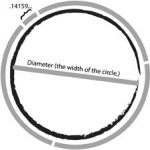# PI Day … 3/14Pi has been calculated to over one trillion digits beyond its decimal point. As an irrational and transcendental number, it will continue infinitely without repetition or pattern. While only a handful of digits are needed for typical calculations, Pi’s infinite nature makes it a fun challenge to memorize, and to computationally calculate more and more digits.

What Is Pi In Math?
Pi (π) is the ratio of a circle’s circumference to its diameter. Pi is a constant number, meaning that for all circles of any size, Pi will be the same.
The diameter of a circle is the distance from edge to edge, measuring straight through the center. The circumference of a circle is the distance around.History of Pi
By measuring circular objects, it has always turned out that a circle is a little more than 3 times its width around. In the Old Testament of the Bible (1 Kings 7:23), a circular pool is referred to as being 30 cubits around, and 10 cubits across. The mathematician Archimedes used polygons with many sides to approximate circles and determined that Pi was approximately 22/7. The symbol (Greek letter π) was first used in 1706 by William Jones. A ‘p’ was chosen for ‘perimeter’ of circles, and the use of π became popular after it was adopted by the Swiss mathematician Leonhard Euler in 1737. In recent years, Pi has been calculated to over one trillion digits past its decimal. Only 39 digits past the decimal are needed to accurately calculate the spherical volume of our entire universe, but because of Pi’s infinite & patternless nature, it’s a fun challenge to memorize, and to computationally calculate more and more digits.

Geometry (Geometry Calculators)
The number pi is extremely useful when solving geometry problems involving circles. Here are some examples:
The area of a circle (Area of a circle calculator).
A = πr2
Where ‘r’ is the radius (distance from the center to the edge of the circle). Also, this formula is the origin of the joke “Pies aren’t square, they’re round!”

The volume of a cylinder (Volume calculator).
V = πr2h
To find the volume of a rectangular prism, you calculate length x width x height. In that case, length x width is the area of one side (the base), which is then multiplied by the height of the prism. Similarly, to find the volume of a cylinder, you calculate the area of the base (the area of the circle), then multiply that by the height (h) of the cylinder.
Last Updated on July 25th, 2018

Source: piday.org

wiki